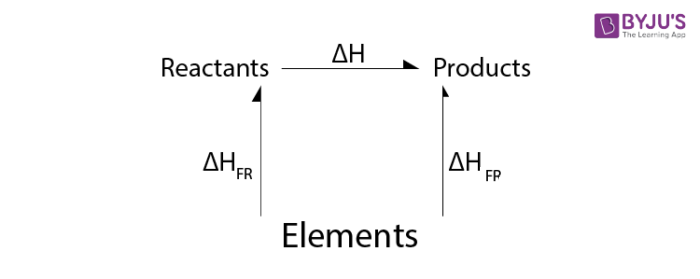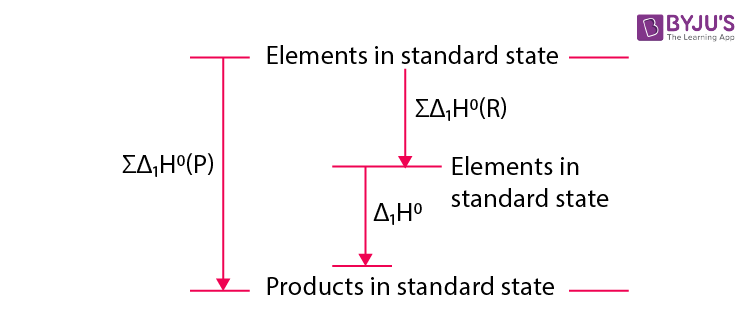# Hess’s Law (Constant Heat Summation)

## What is Hess’s Law?

Hess’s law is one of the important outcomes of the first law of thermodynamics.

The enthalpy change in a chemical or physical process is the same whether the process is carried out in one step or in several steps.

The law for constant heat summation was derived in the year 1840, a Swiss-born Russian chemist and physician, Germain Hess, derived a relationship in thermochemistry for calculating the standard reaction enthalpy for multistep reactions. It basically exploits the properties of state functions, that is the value of state functions does not depend on the path taken for formation or dissociation rather it depends only on state at the moment (temperature, pressure, volume, etc).As we know enthalpy is a state function, hence, it is independent of the path taken to reach the final state starting from the initial state. According to Hess’s law, for a multistep reaction, the standard reaction enthalpy is independent of the pathway or number of steps taken, rather it is the sum of standard enthalpies of intermediate reactions involved at the same temperature.

The purpose of Hess’s law is to measure the enthalpies of neutralization for several acid-base reactions, then use that information and Hess’s law to determine the reaction enthalpies for two salts in aqueous solution.

## Application of Hess’s Law

Let us look at some practical areas where Hess’s law is applied, for example, the formation of Sulphur Trioxide gas from Sulphur is a multistep reaction involving the formation of Sulphur Dioxide gas. Let us find out the standard reaction enthalpy for the formation of Sulphur Trioxide gas from Sulphur.

Step 1: Formation of Sulphur Dioxide gas

$$\begin{array}{l} S + O_2 \rightarrow SO_2 ~~~~~~~~~~~~~~~~~~~\triangle H_1 = -70.96KCal/mol\end{array}$$

Step 2: Conversion of Sulphur Dioxide gas to Sulphur Trioxide gas

$$\begin{array}{l}SO_2 + \frac{1}{2} O_2 \rightarrow SO_3 ~~~~~~~~~~~~ \triangle H_2= – 23.49 KCal/mol\end{array}$$

Standard reaction enthalpy as per Hess’s Law:

$$\begin{array}{l}\triangle H_R = \triangle H_1 + \triangle H_2 = (-70.96) + (-23.49) = -94.95 KCal/mol\end{array}$$

Net reaction:

$$\begin{array}{l}S + \frac{3}{2} O_2 \rightarrow SO_3 ~~~~~~~~~~~~~~~ \triangle H_R = -94.95 KCal/mol\end{array}$$

Hence, in simple words we can state:

$$\begin{array}{l}\triangle H_R = \triangle H_1 + \triangle H_2 + \triangle H_3 + \triangle H_4 +…………….\end{array}$$

## Determination of Enthalpy of Formation

There are many compounds such as C6H6, Co, C2H6 etc, whose direct synthesis from their constituent elements is not possible. Their ΔH values can be determined indirectly by Hess’s law.

As with enthalpy, Hess’s Law can be used to determine other state functions such as free energy and entropy, for example, Bordwell thermodynamic cycle which takes advantage of easily measured equilibriums and Redox potentials to determine experimentally inaccessible Gibbs free energy values.

$$\begin{array}{l}\triangle G_{(reaction)} = \sum \triangle G_{(product)} – \sum \triangle G_{(reactants)}\end{array}$$

As entropy can be measured as an absolute value, hence in case of entropy there is no need to use the entropy of formation.

$$\begin{array}{l}\triangle S_{(reaction)} = \sum S_{(products)} – \sum S_{(reactants)}\end{array}$$

## Calculation of Standard Enthalpies of Reaction

From the standard enthalpies of formation of reactants and products, the standard enthalpy of reaction can be calculated using Hess’s law. In general, the Hess’s law cycle representing the formation of reactants and products from their respective elements in the standard state is considered asAccording to Hess’s law,

$$\begin{array}{l}\sum \Delta_{1} H^{o}(P)=\sum \Delta_{1} H^{o}(R)+\Delta_{R}H^{o}\end{array}$$
$$\begin{array}{l}\Delta_{R}H^{o} = \sum \Delta_{1} H^{o}(P)-\sum \Delta_{1} H^{o}(R)\end{array}$$
$$\begin{array}{l}= Sum\ of\ standard\ enthalpies\ of\ formation\ of\ products\ -\ Sum\ of\ standard\ enthalpies\ of\ formation\ of\ reactants\end{array}$$

## Solved Example

Question:

Calculate the standard enthalpy change for a reaction
CO2(g) + H2(g) → CO(g) + H2O(g)
Given that ΔrHo for CO2(g), CO(g) and H2O(g) as -393.5, -110.5 and 241.8kJ/mol respectively.

Solution:

ΔrHo for the reaction is given as

$$\begin{array}{l}\Delta_{r}H^{o} = \sum \Delta_{f} H^{o}(Products)-\sum \Delta_{f} H^{o}(Reactants)\end{array}$$
$$\begin{array}{l}= [\Delta_{f} H^{o}(H_{2}O)+ \Delta_{f} H^{o}(CO)]-[\Delta_{f} H^{o}(CO_{2})+ \Delta_{f} H^{o}(H_{2})]\end{array}$$

Substituting the given values, we get

$$\begin{array}{l}\Delta_{r} H^{o}=[-241.8-110.5]-[-393.5+0]=-352.3+393.5\end{array}$$

= + 41.2 kJ

## Frequently Asked Questions – FAQs

Q1

### What is the basis of Hess’s law?

Hess’s law states that the increase in enthalpy in a chemical reaction i.e., reaction heat at constant pressure is independent of the process between the initial and final states.

Q2

### What means entropy?

It generally refers to the idea that, inevitably, everything in the universe transitions from order to chaos, and entropy is the measure of that transition. The term entropy has its origins in Greek entropy, meaning “a change toward” or “transformation.”

Q3

### Why is Hess law important?

Hess’s law allows the enthalpy shift (even if it can not be determined directly) to be estimated for a reaction. This is achieved by carrying out simple algebraic operations based on the chemical equation of reactions using values previously defined for the formation of enthalpies.

Q4

### What does Hess’s law state?

The Hess’s law states that in a chemical reaction, the change of enthalpy (i.e. the heat of reaction under constant pressure) is independent of the direction between the original and final states. The law of Hess requires the change in enthalpy ( Δ H) for a reaction to be determined even though it can not be directly measured.

Q5

### What is Hess law explain with example?

Hess’s Constant Heat Summation Law (or only Hess’s Law) states that the overall change in enthalpy for the solution is the sum of all changes independent of the different phases or steps of a reaction. This rule is a discovery that enthalpy is a part of the state.